The plot_normality() visualize distribution information for normality test of the numerical(INTEGER, NUMBER, etc.) column of the DBMS table through tbl_dbi.

# S3 method for tbl_dbi
plot_normality(
.data,
...,
in_database = FALSE,
collect_size = Inf,
left = c("log", "sqrt", "log+1", "1/x", "x^2", "x^3", "Box-Cox", "Yeo-Johnson"),
right = c("sqrt", "log", "log+1", "1/x", "x^2", "x^3", "Box-Cox", "Yeo-Johnson"),
col = "steelblue",
typographic = TRUE,
base_family = NULL
)

## Arguments

.data a tbl_dbi. one or more unquoted expressions separated by commas. You can treat variable names like they are positions. Positive values select variables; negative values to drop variables. If the first expression is negative, plot_normality() will automatically start with all variables. These arguments are automatically quoted and evaluated in a context where column names represent column positions. They support unquoting and splicing. See vignette("EDA") for an introduction to these concepts. Specifies whether to perform in-database operations. If TRUE, most operations are performed in the DBMS. if FALSE, table data is taken in R and operated in-memory. Not yet supported in_database = TRUE. a integer. The number of data samples from the DBMS to R. Applies only if in_database = FALSE. character. Specifies the data transformation method to draw the histogram in the lower left corner. The default is "log". character. Specifies the data transformation method to draw the histogram in the lower right corner. The default is "sqrt". a color to be used to fill the bars. The default is "steelblue". logical. Whether to apply focuses on typographic elements to ggplot2 visualization. character. The name of the base font family to use for the visualization. If not specified, the font defined in dlookr is applied. (See details)

## Details

The scope of the visualization is the provide a distribution information. Since the plot is drawn for each variable, if you specify more than one variable in the ... argument, the specified number of plots are drawn.

The argument values that left and right can have are as follows.:

• "log" : log transformation. log(x)

• "log+1" : log transformation. log(x + 1). Used for values that contain 0.

• "sqrt" : square root transformation.

• "1/x" : 1 / x transformation

• "x^2" : x square transformation

• "x^3" : x^3 square transformation

• "Box-Cox" : Box-Box transformation

• "Yeo-Johnson" : Yeo-Johnson transformation

## Distribution information

The plot derived from the numerical data visualization is as follows.

• histogram by original data

• q-q plot by original data

• histogram by log transfer data

• histogram by square root transfer data

The base_family is selected from "Roboto Condensed", "Liberation Sans Narrow", "NanumSquare", "Noto Sans Korean". If you want to use a different font, use it after loading the Google font with import_google_font().

plot_normality.data.frame, plot_outlier.tbl_dbi.

## Examples

# \donttest{
library(dplyr)

# connect DBMS
con_sqlite <- DBI::dbConnect(RSQLite::SQLite(), ":memory:")

# copy heartfailure to the DBMS with a table named TB_HEARTFAILURE
copy_to(con_sqlite, heartfailure, name = "TB_HEARTFAILURE", overwrite = TRUE)

# Using pipes ---------------------------------
# Visualization of all numerical variables
con_sqlite %>%
tbl("TB_HEARTFAILURE") %>%
plot_normality()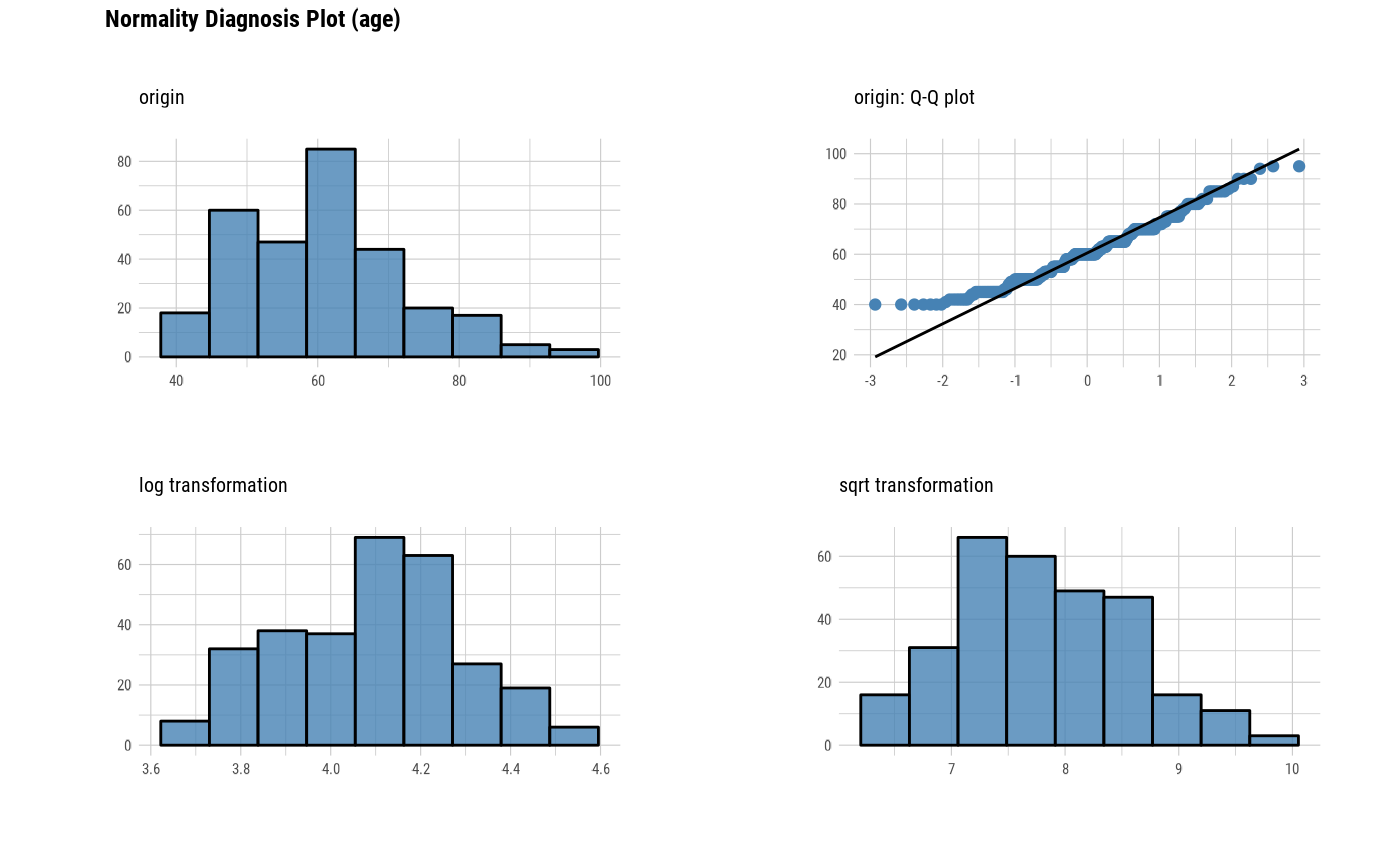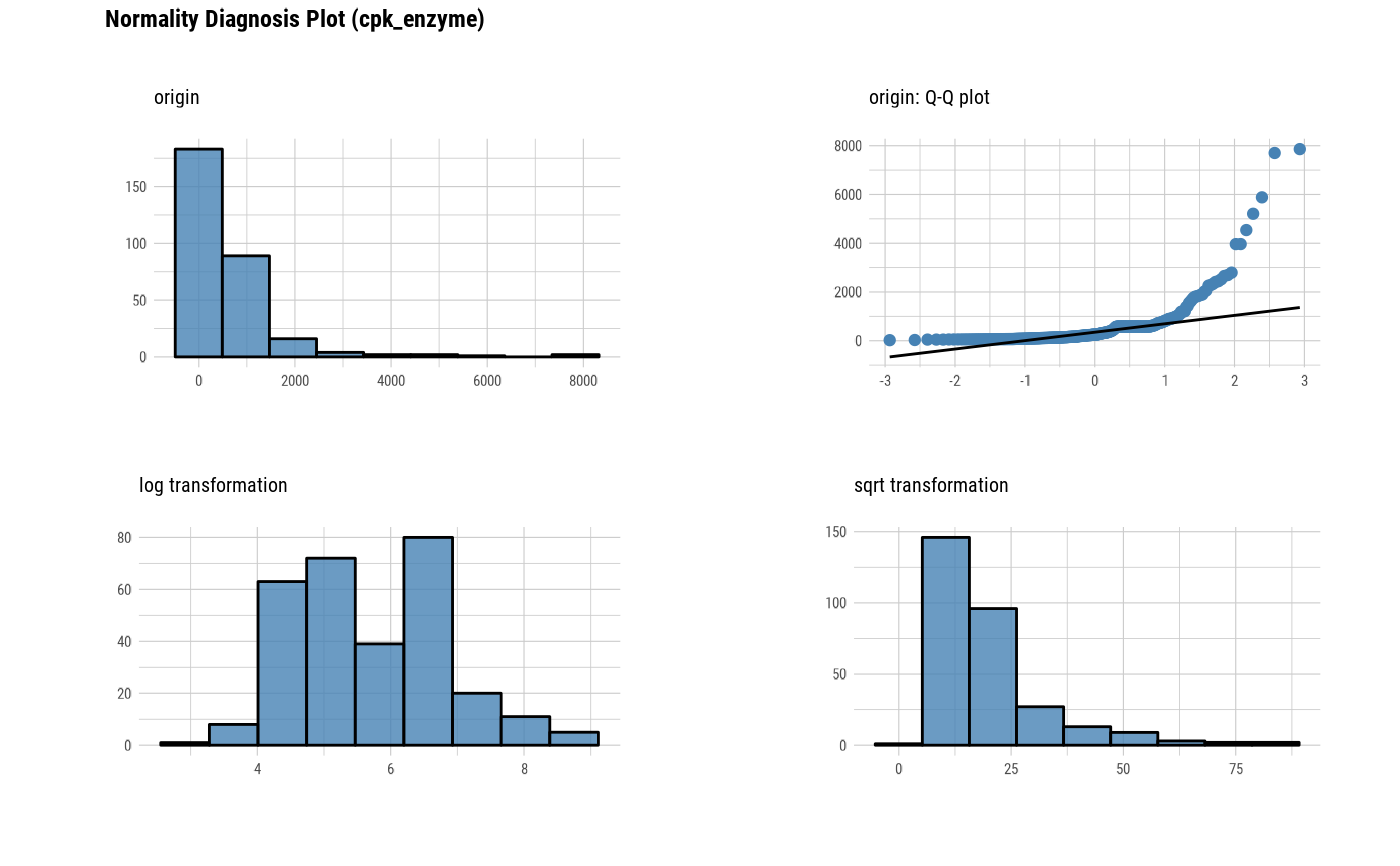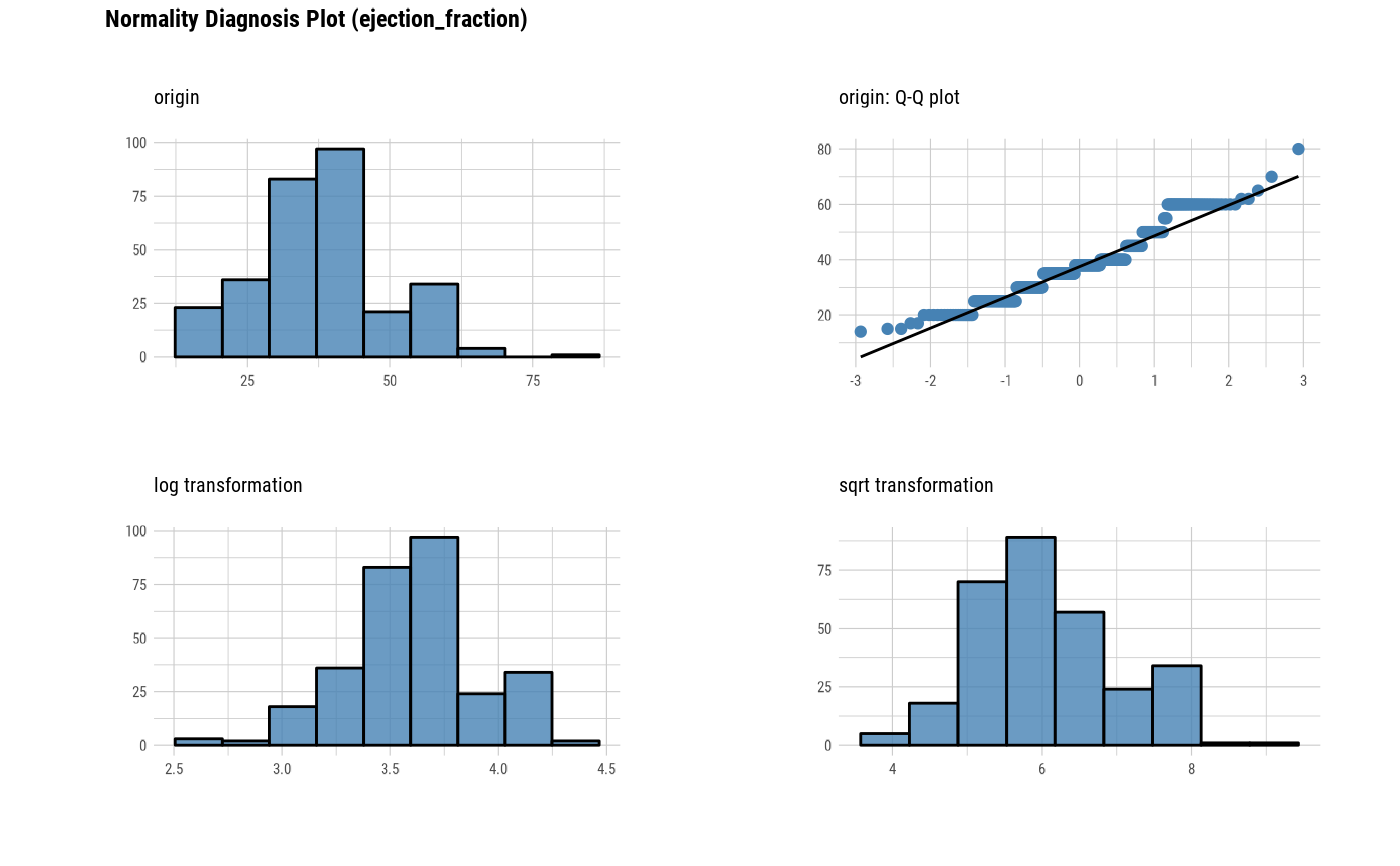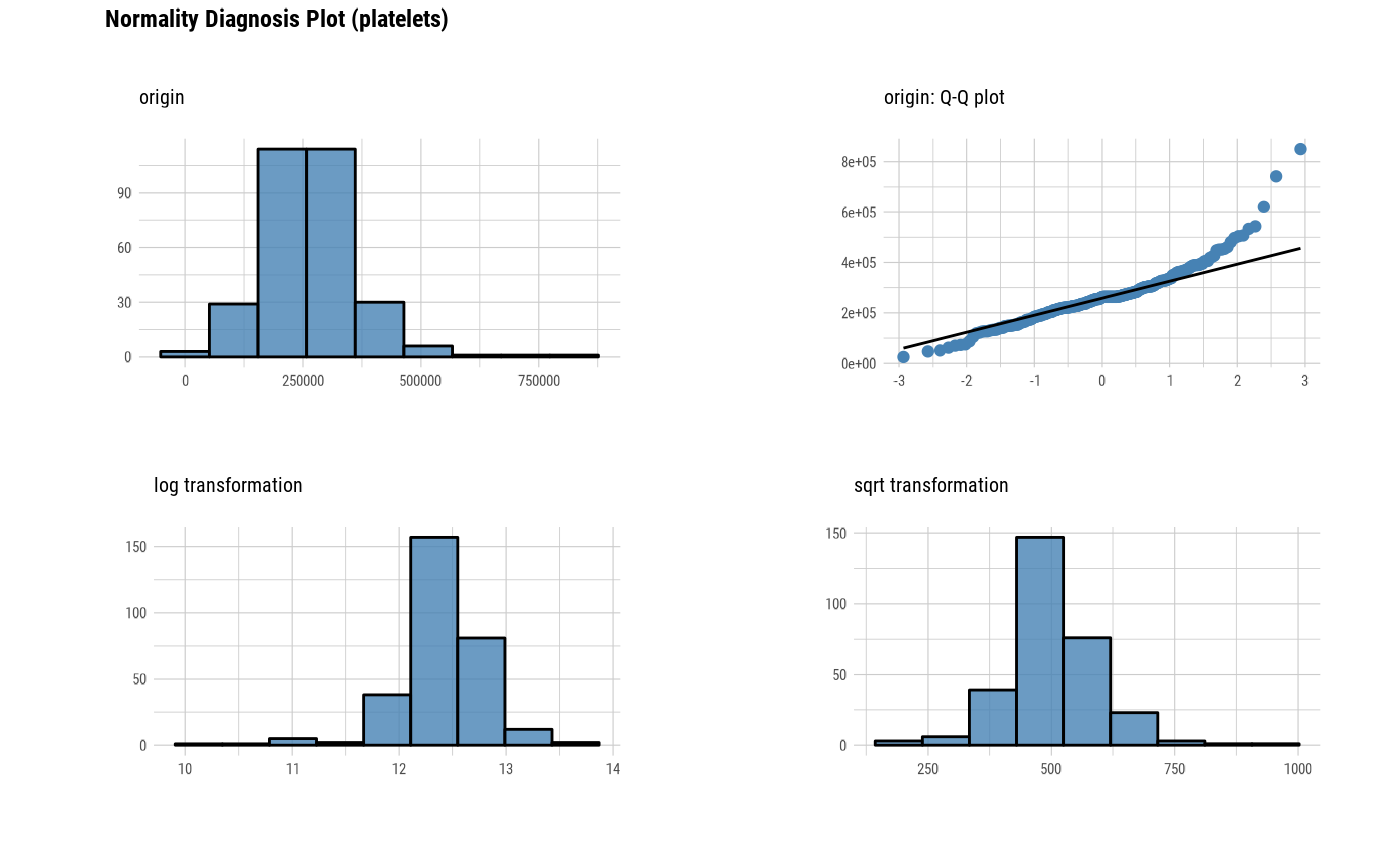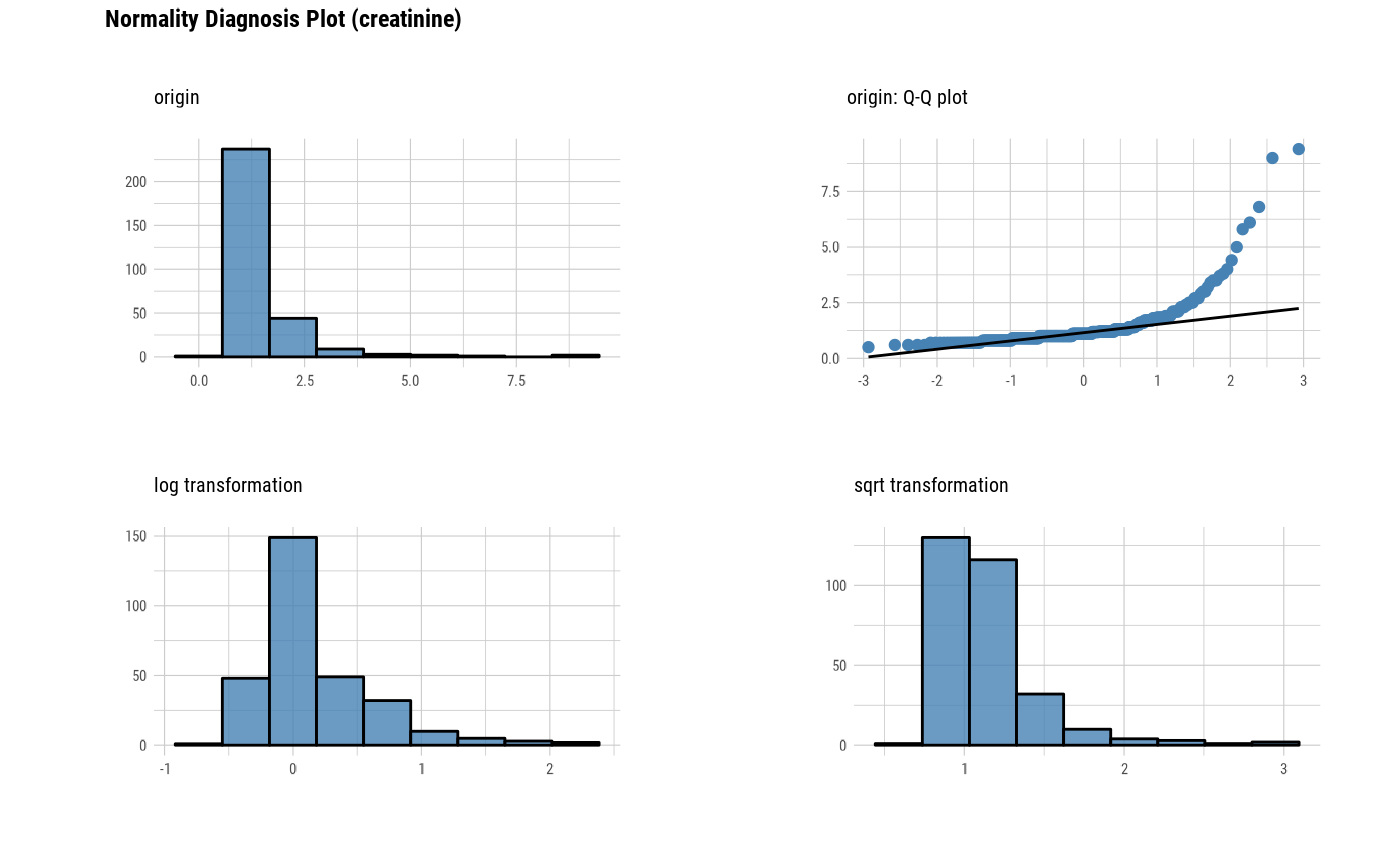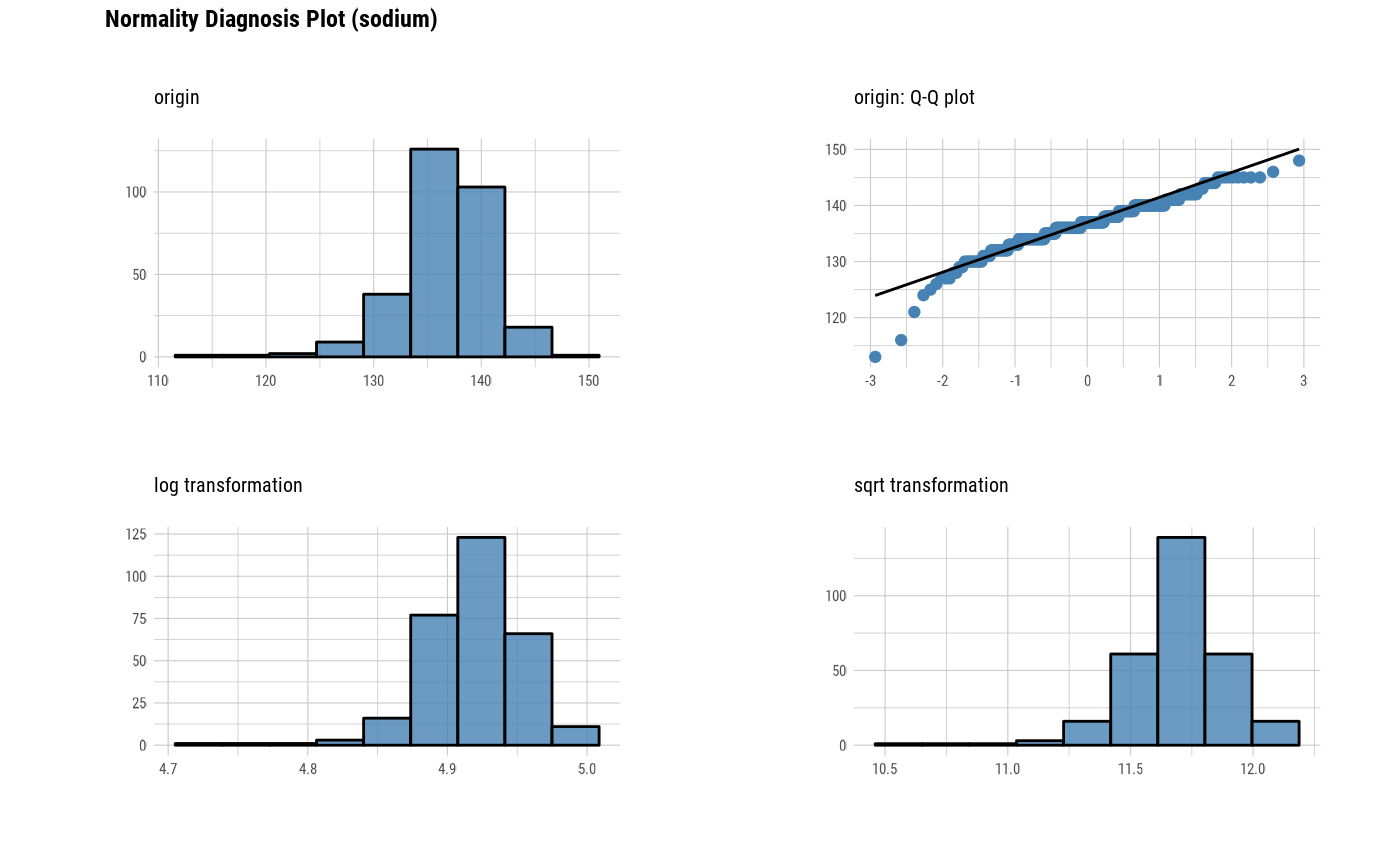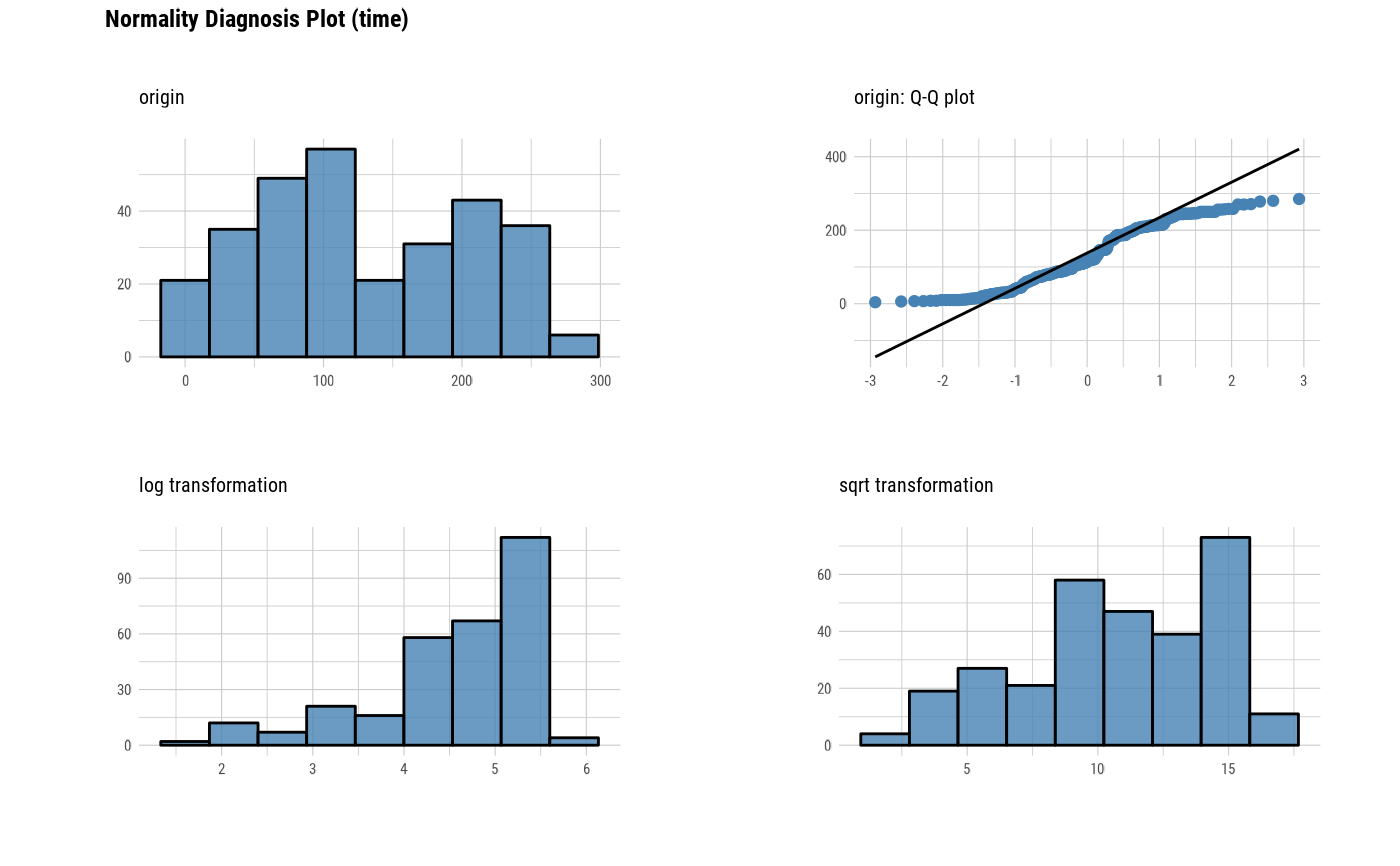# Positive values select variables, and In-memory mode and collect size is 200
con_sqlite %>%
tbl("TB_HEARTFAILURE") %>%
plot_normality(platelets, sodium, collect_size = 200)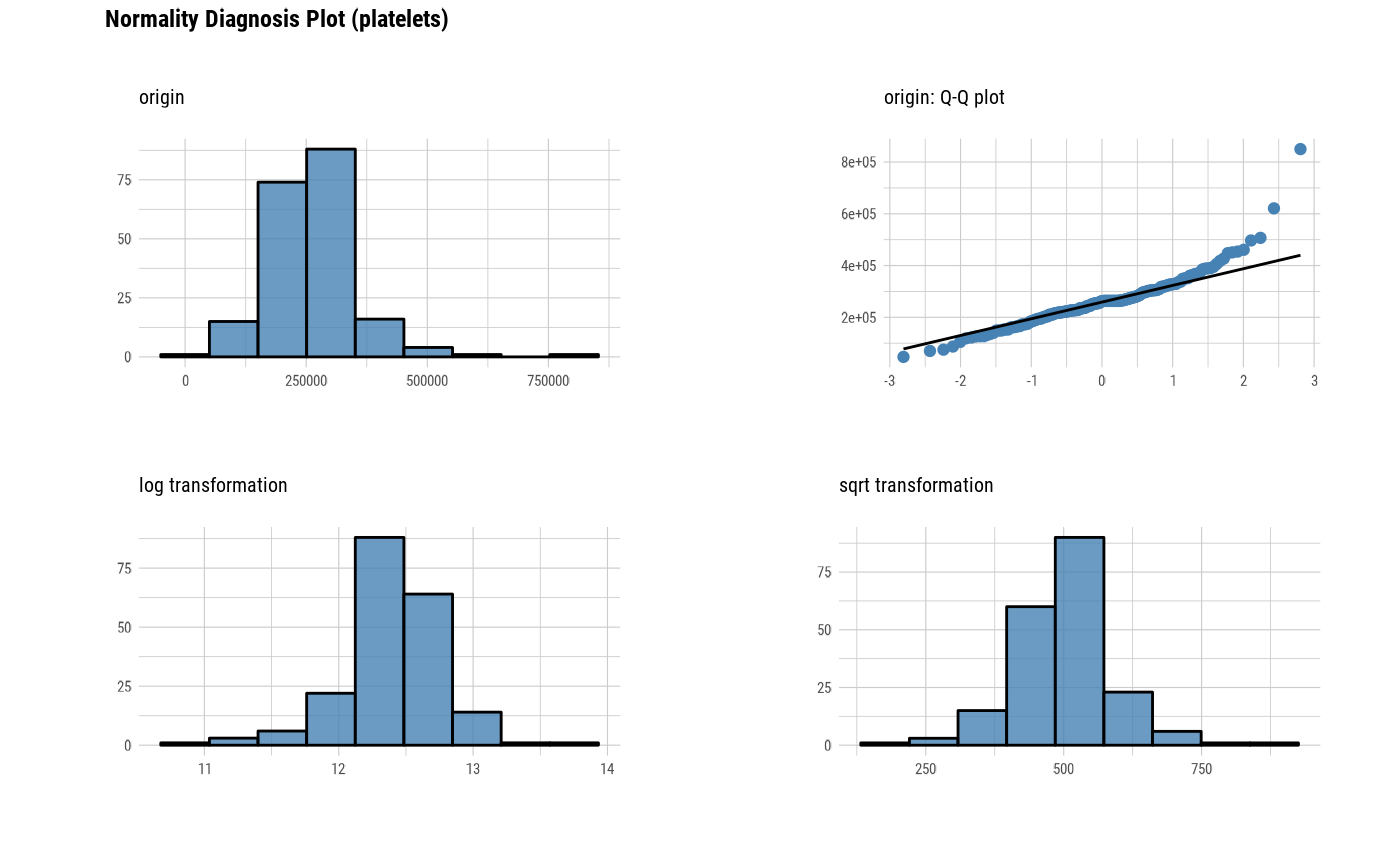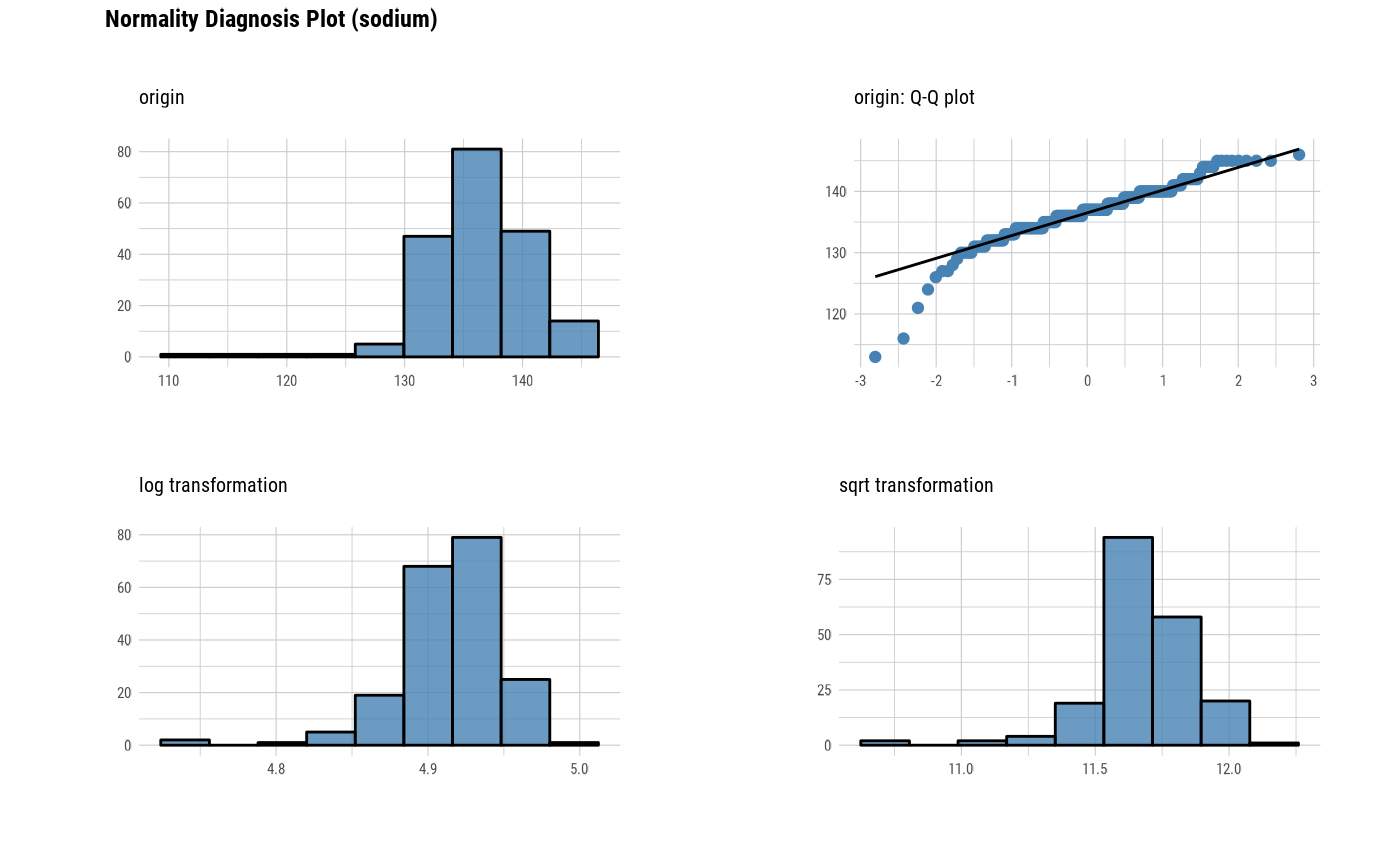# Positions values select variables
con_sqlite %>%
tbl("TB_HEARTFAILURE") %>%
plot_normality(1)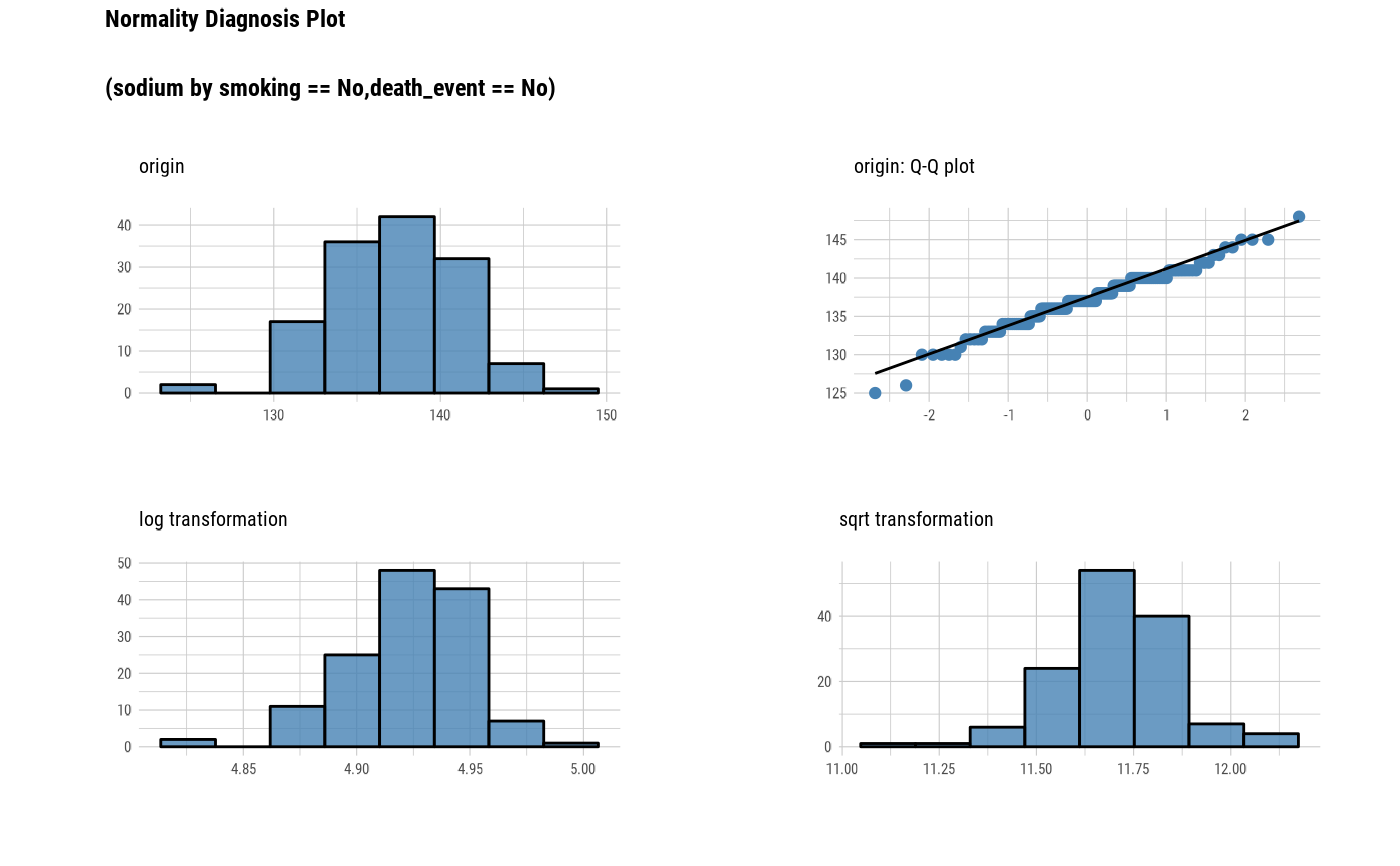# Not allow the typographic elements
con_sqlite %>%
tbl("TB_HEARTFAILURE") %>%
plot_normality(1, typographic = FALSE)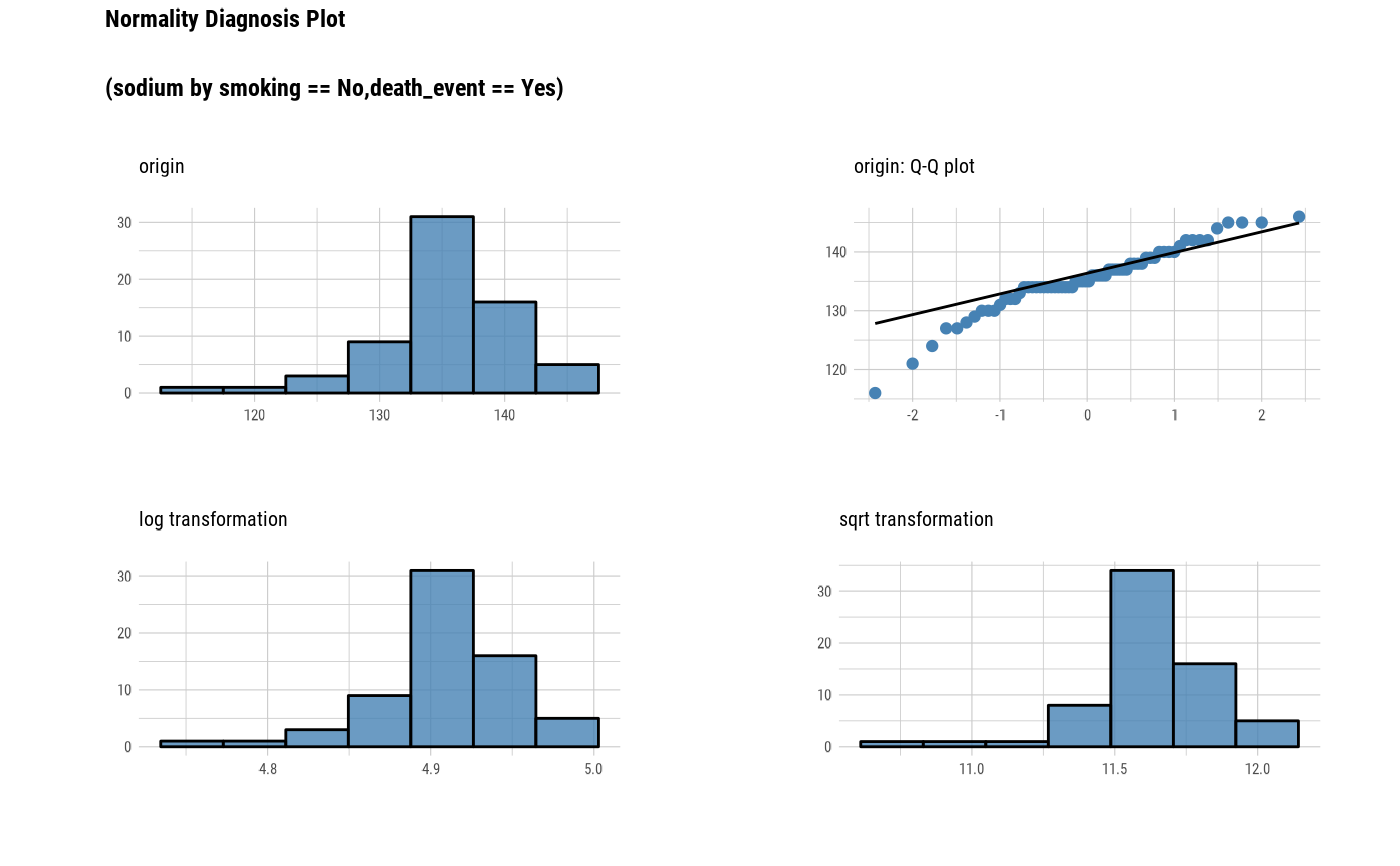# Using pipes & dplyr -------------------------
# Plot 'sodium' variable by 'smoking' and 'death_event'
con_sqlite %>%
tbl("TB_HEARTFAILURE") %>%
group_by(smoking, death_event) %>%
plot_normality(sodium)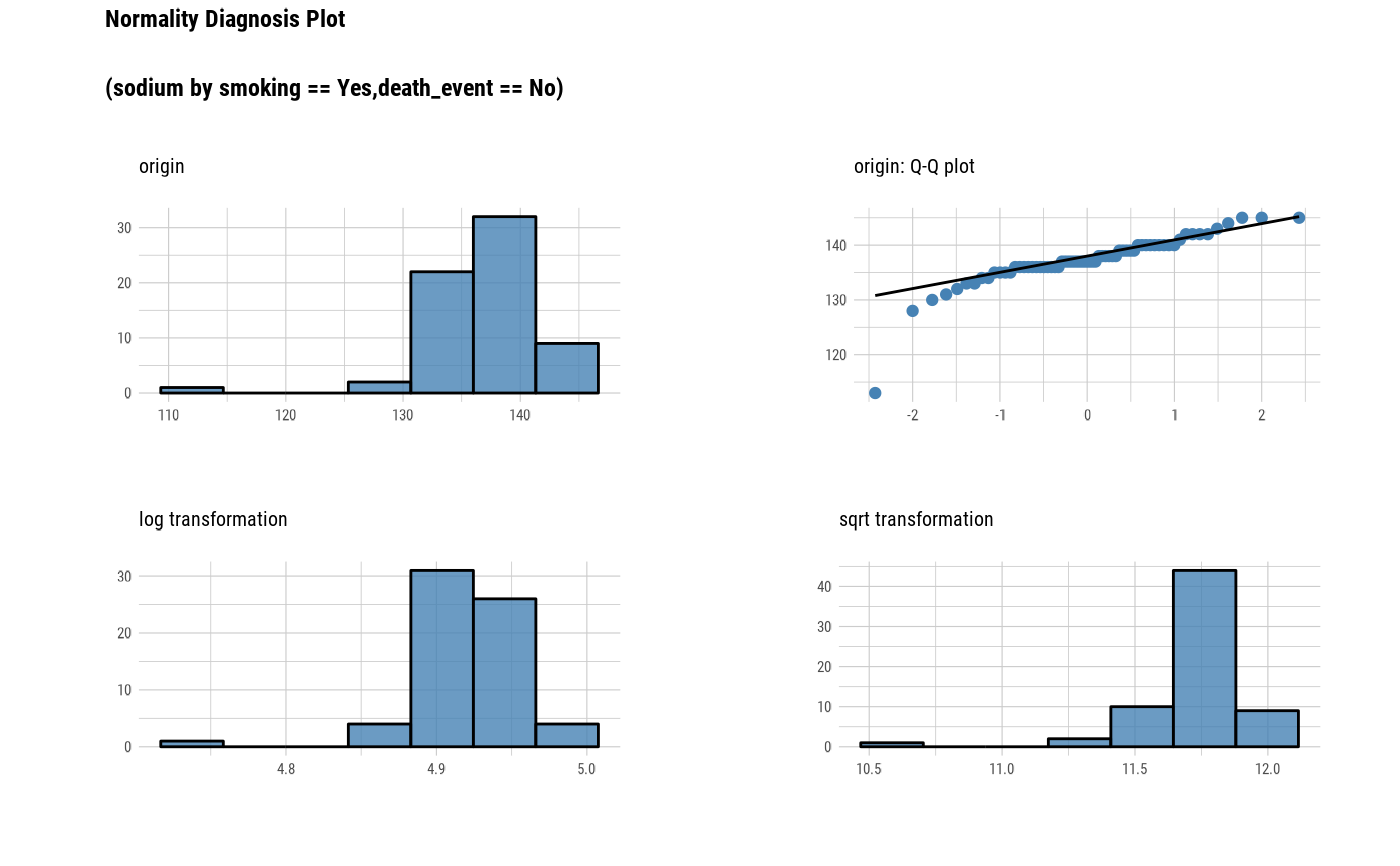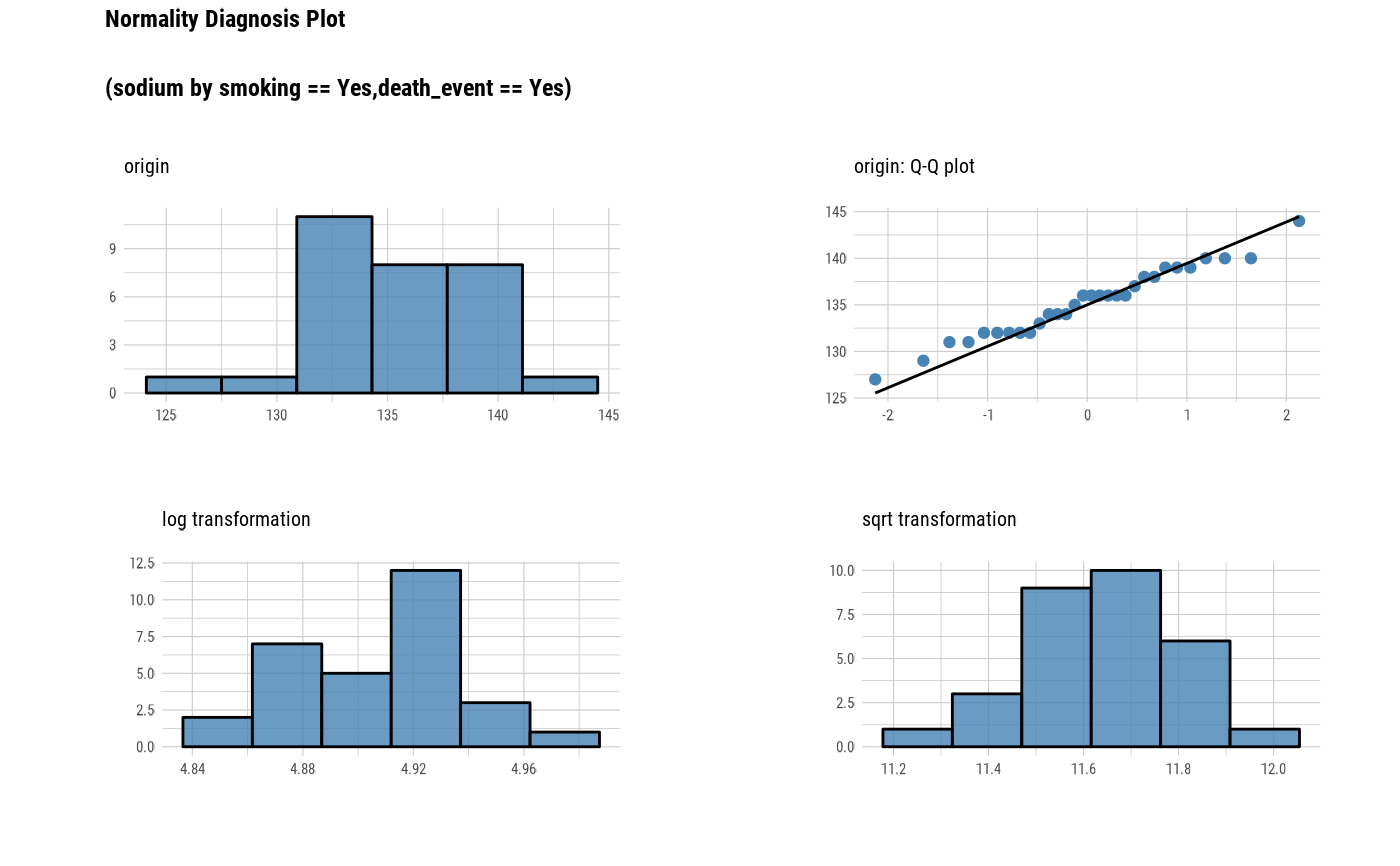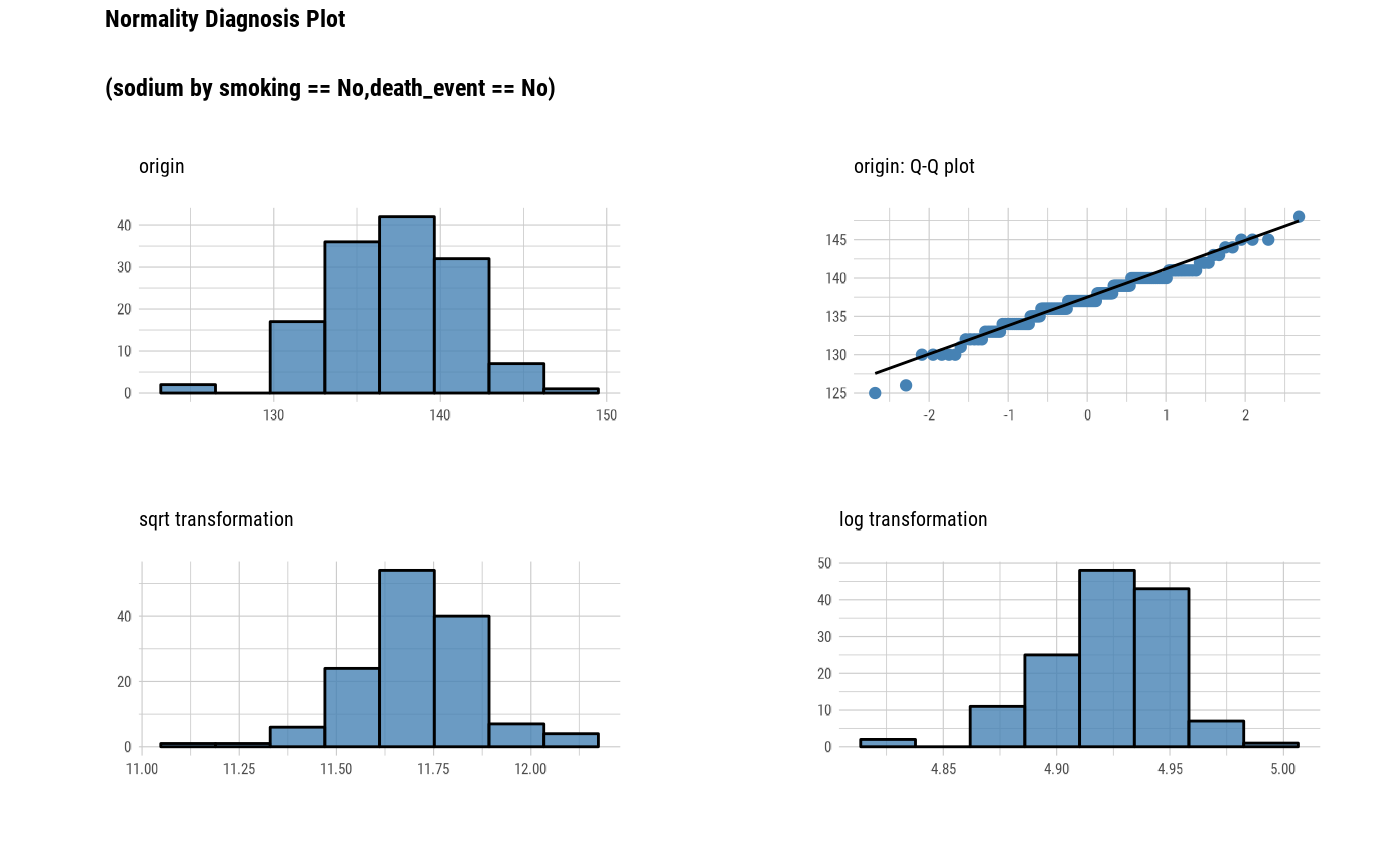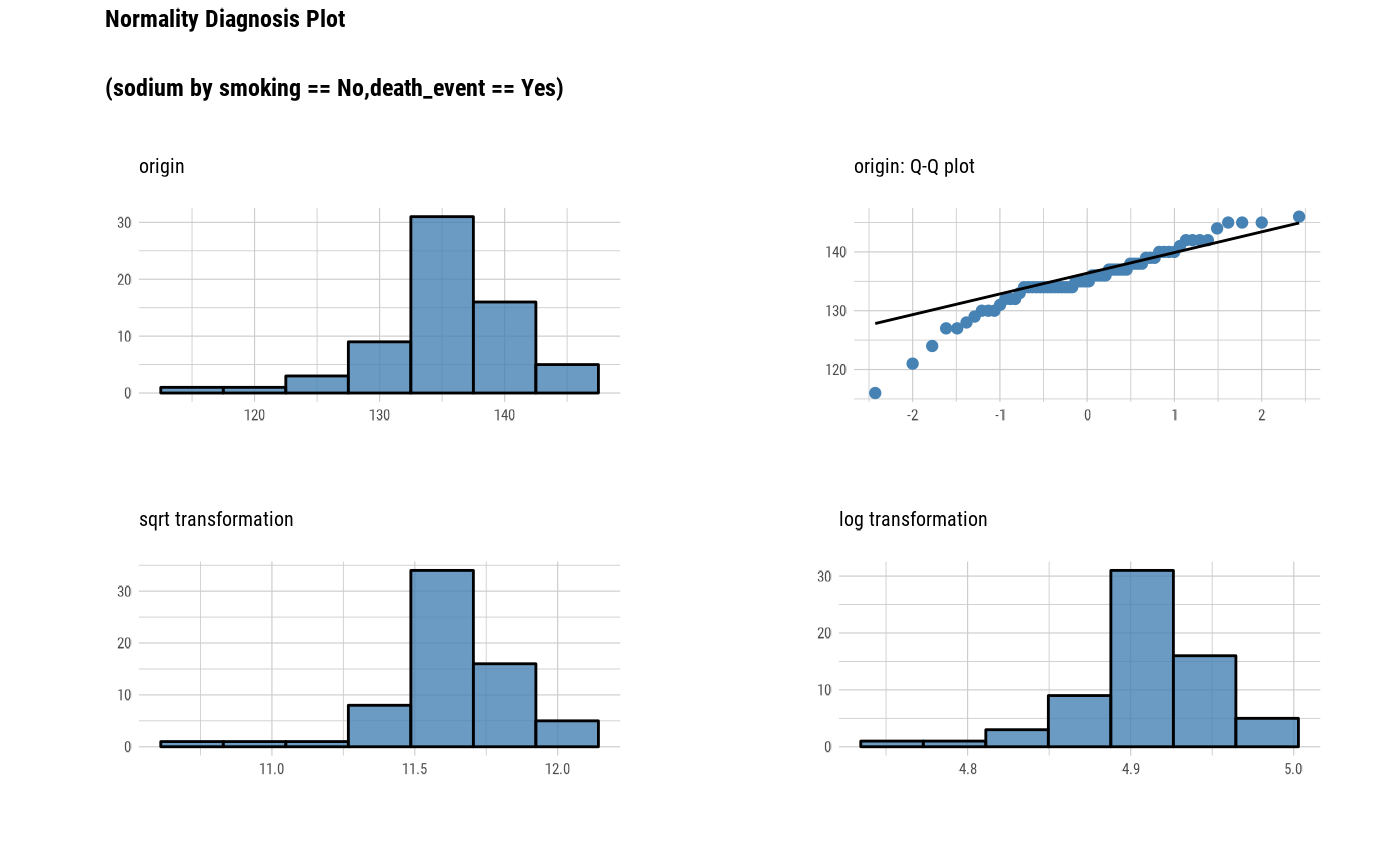# Plot using left and right arguments
con_sqlite %>%
tbl("TB_HEARTFAILURE") %>%
group_by(smoking, death_event) %>%
plot_normality(sodium, left = "Box-Cox", right = "log")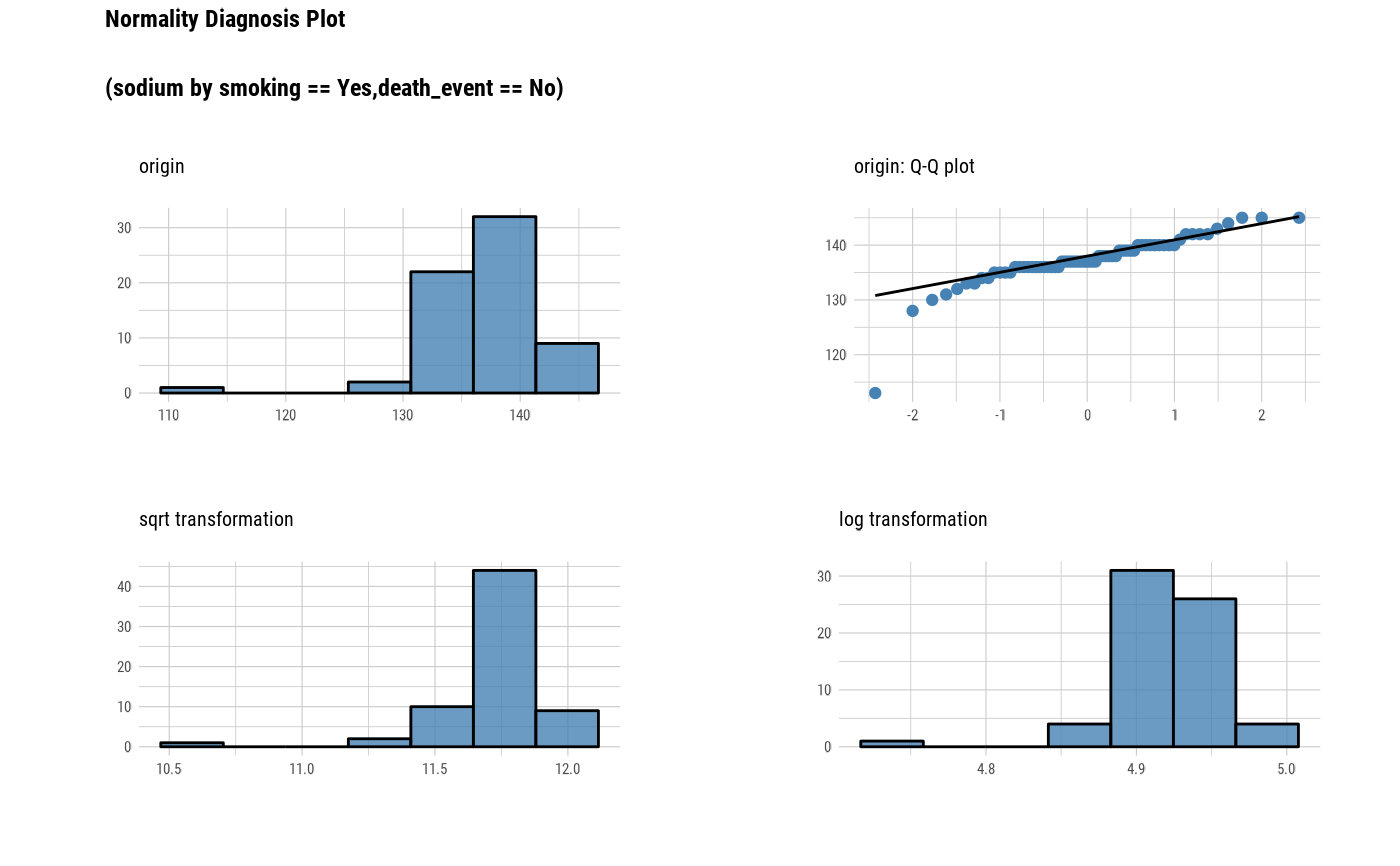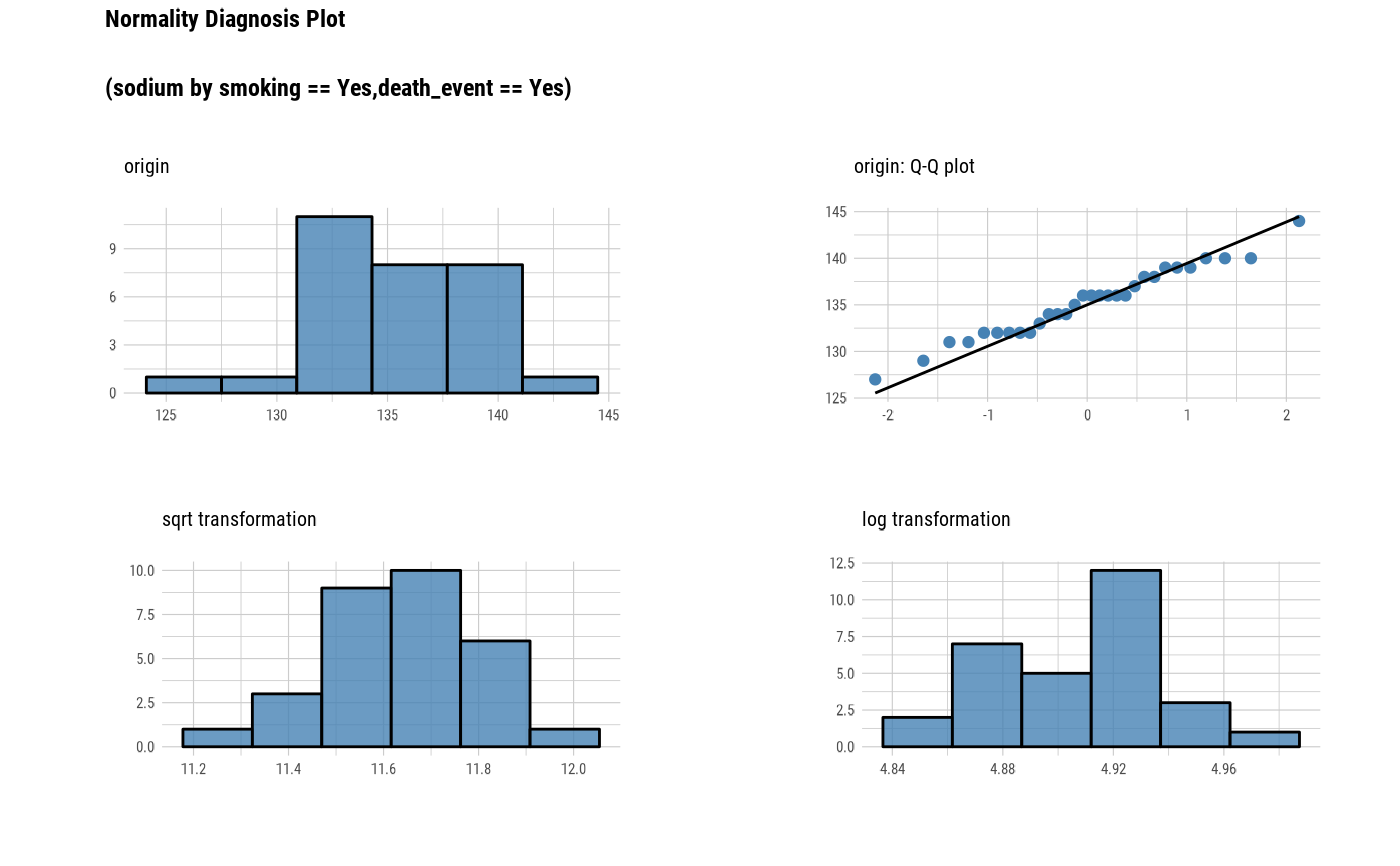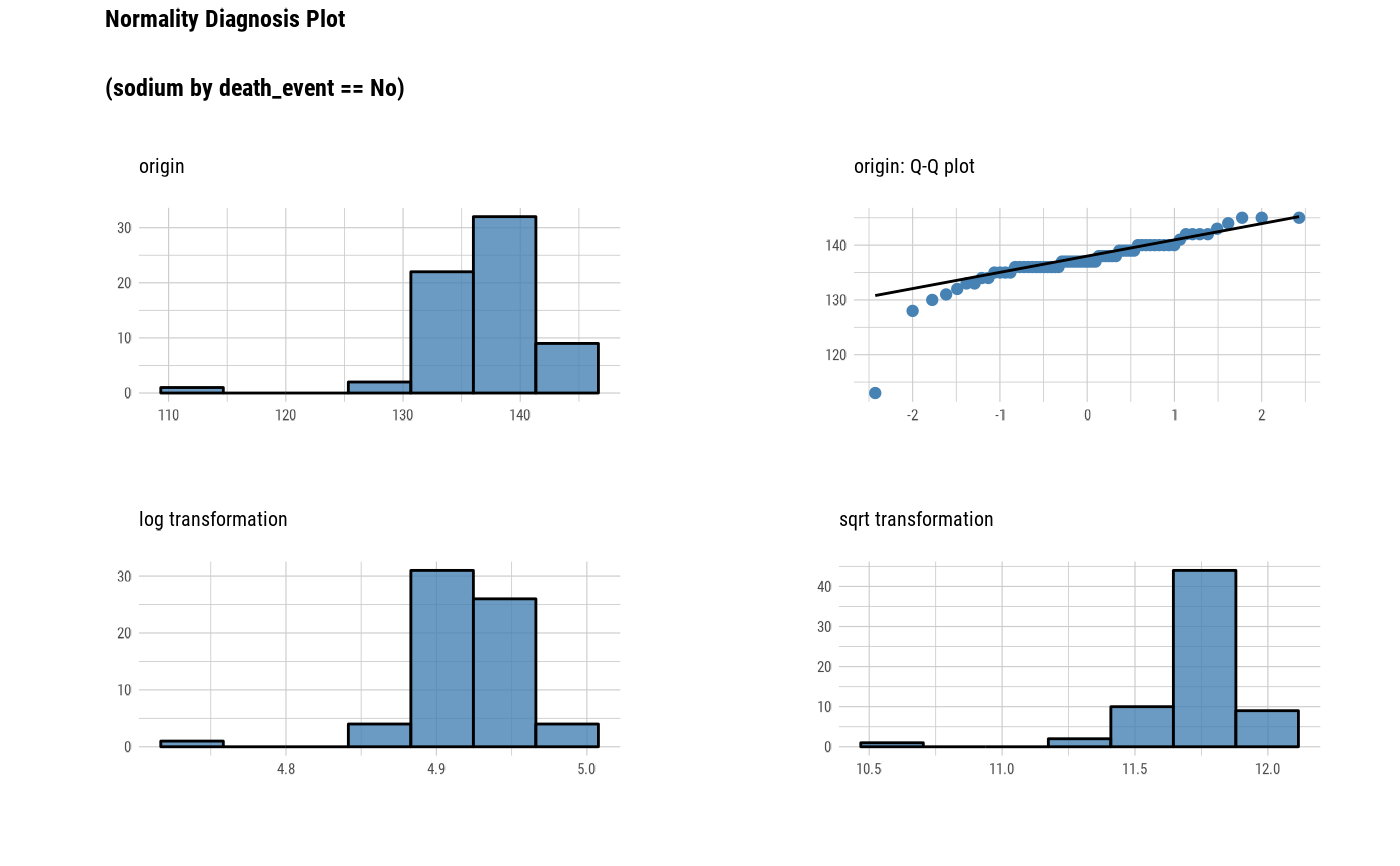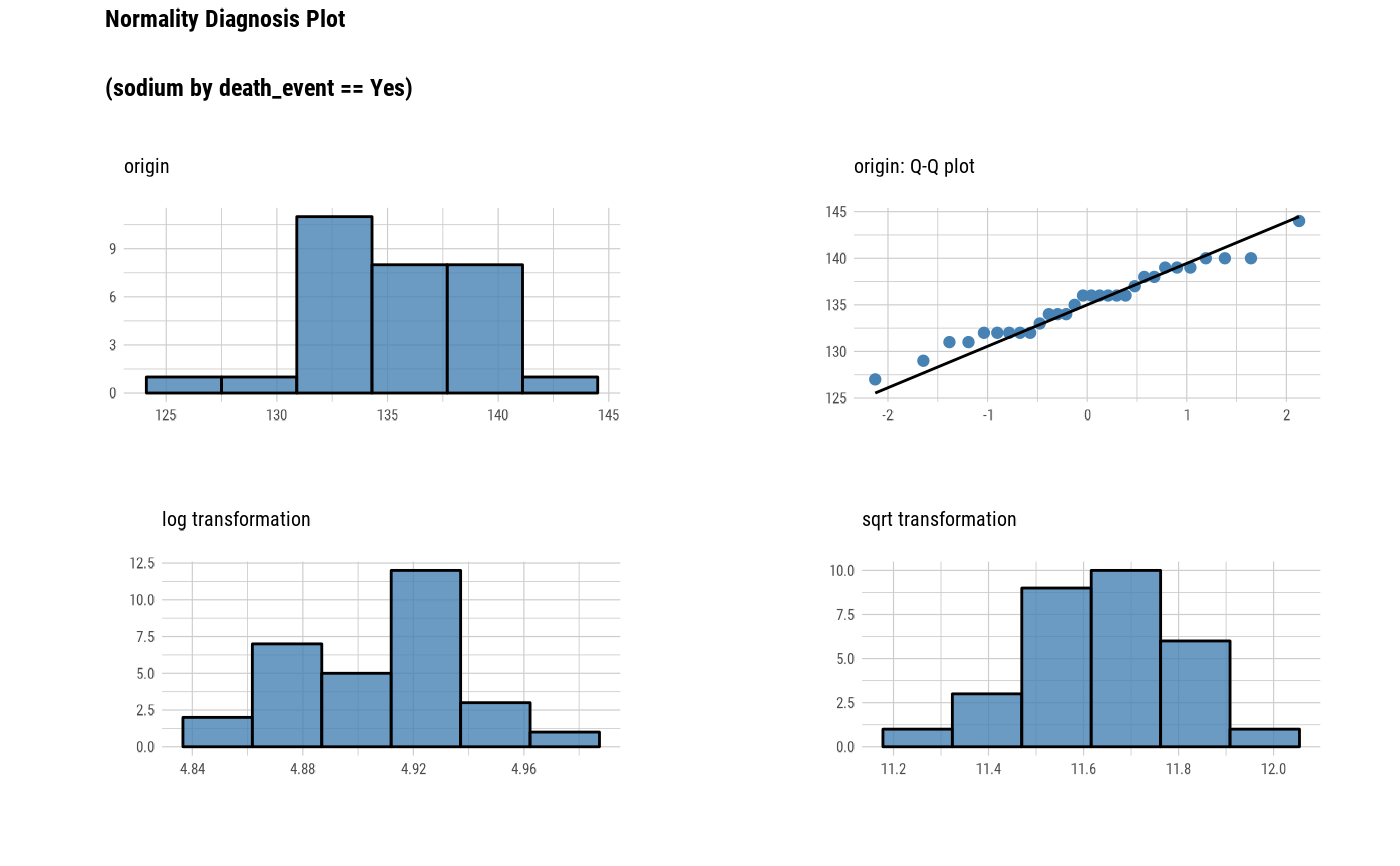# extract only those with 'smoking' variable level is "Yes",
# and plot 'sodium' by 'death_event'
con_sqlite %>%
tbl("TB_HEARTFAILURE") %>%
filter(smoking == "Yes") %>%
group_by(death_event) %>%
plot_normality(sodium)# Disconnect DBMS
DBI::dbDisconnect(con_sqlite)
# }